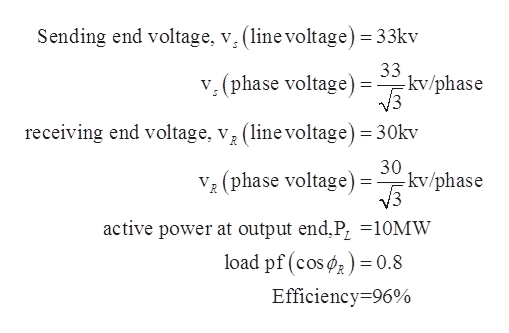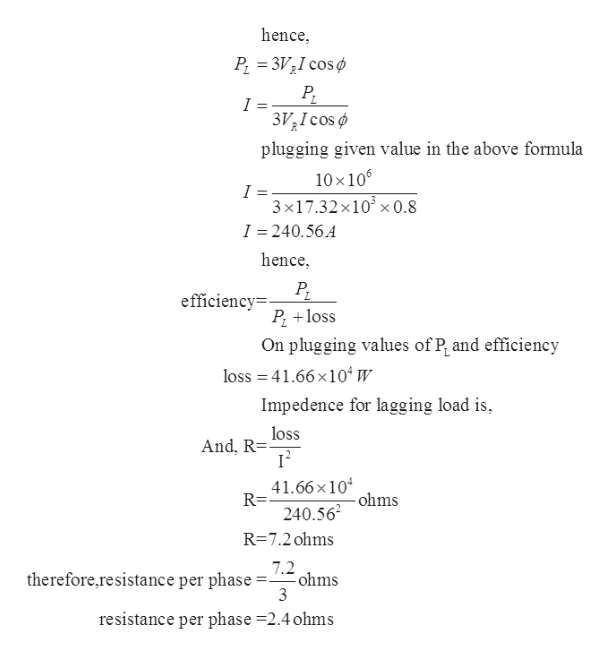# A short 3 phase transmission line, connected to a 33kV, 50 Hz generating station at the sending end is required to supply a load of 10Megawatts at 0.8 power factor lagging, 30kV at the receiving end. If the minimum transmission line efficiency is to be limited to 96%, estimate the per phase values of resistance and inductance of the line.

Question

A short 3 phase transmission line, connected to a 33kV, 50 Hz generating station at the sending end is required to supply a load of 10Megawatts at 0.8 power factor lagging, 30kV at the receiving end. If the minimum transmission line efficiency is to be limited to 96%, estimate the per phase values of resistance and inductance of the line.

check_circleExpert Solution
Step 1

As given in the questionhelp_outlineImage TranscriptioncloseSending end voltage, v, (linevoltage) 33kv 33 -kv/phase v. (phase voltage) - receiving end voltage, v (line voltage) 3Okv 30 -kv/phase (phase voltage) active power at output end,P, 10MW load pf (cosø0.8 Efficiency-96% fullscreen
Step 2

To calculate resistance steps is shown belowhelp_outlineImage Transcriptionclosehence P 3V2I cos 3I cos plugging given value in the above formula 10x10 I = 3 x17.32x10x 0.8 I 240.56.4 hence efficiency= P loss On plugging values of P and efficiency loss 41.66x10w Impedence for lagging load is loss And, R= 41.66x10 R= ohms 240.562 R=7.2 ohms 7.2 therefore,resistance per phase ohms 3 resistance per phase 2.4 ohms fullscreen
Step 3

Now to calculate reactance s...

### Want to see the full answer?

See Solution

#### Want to see this answer and more?

Solutions are written by subject experts who are available 24/7. Questions are typically answered within 1 hour*

See Solution
*Response times may vary by subject and question
Tagged in

### Electrical Engineering